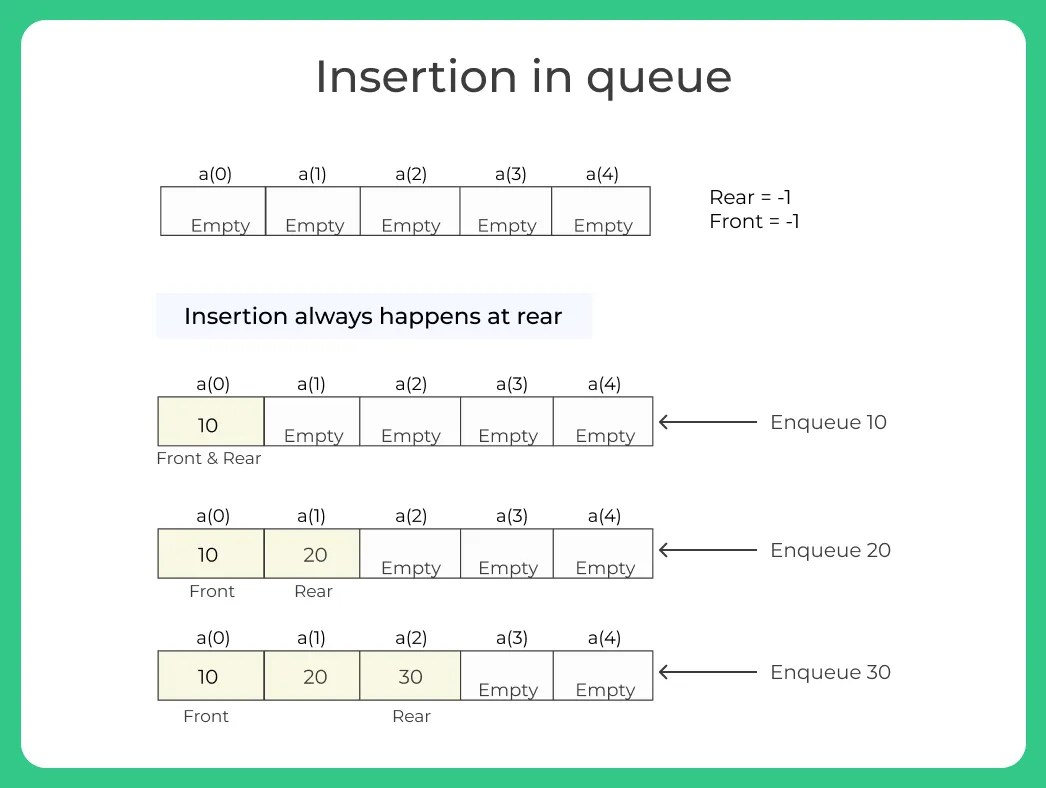# Insertion in a Queue in Java## How to insert an element in a Queue using JAVA programming?

Insertion in a queue in java is a simple operation that we can perform on Queue Data Structure. Insertion in a Queue is also known as Enqueue operation when we are talking in respect with Queues. There are several different types of Queues, which we learn afterwards. In this article lets learn how to code a C++ Program for Inserting an Element in a Queue

## Steps to insert an element in a Queue in Java​​

1. In the main function we will take size of queue as user input.
2. And then we will initialize a for loop that will iterate till the size of queue.
3. On every iteration we will ask the data from the user and will insert that data in the queue using enqueue function.
4. In the enqueue function first we will increase the value of rear by one on every successful iteration.
5. And will insert data at rear of the queue.
6. Later we will use the display function to display the data that is present in the queue.## Algorithm to insert an element in a Queue in Java

• if(rear == MAX-1)
• Print “Queue is full”
• else
• rear = rear + 1
• queue[rear]=data
• if(front== -1)
• front=0

## Java programming code for insertion in a Queue(Enqueue)

Run
```class Queue {
int front, rear, size;
int capacity;
int array[];

public Queue(int capacity)
{
this.capacity = capacity;
front = this.size = 0;
rear = capacity - 1;
array = new int[this.capacity];
}

// Queue is full when size becomes
// equal to the capacity
boolean isFull(Queue queue)
{
return (queue.size == queue.capacity);
}

// Queue is empty when size is 0
boolean isEmpty(Queue queue)
{
return (queue.size == 0);
}

// Method to add an item to the queue.
// It changes rear and size
void enqueue(int item)
{
if (isFull(this))
return;
this.rear = (this.rear + 1)
% this.capacity;
this.array[this.rear] = item;
this.size = this.size + 1;
System.out.println(item
+ " enqueued to queue");
}

public void disp() /* function to display the elements of the queue */ {

System.out.print("\nThe elements in the queue are:");
if(front == -1) {
System.out.print("\nQueue is Empty");
}
else {
for(int i = front; i <= rear; i++) {
System.out.print(array[i] + "  ");
}
}
System.out.println();
}

}

// Driver class
public class Main {
public static void main(String[] args)
{
Queue queue = new Queue(1000);

queue.enqueue(10);
queue.enqueue(20);
queue.enqueue(30);
queue.enqueue(40);

queue.disp();

System.out.println();

}
}
```
``` Output:
10 enqueued to queue
20 enqueued to queue
30 enqueued to queue
40 enqueued to queue

The elements in the queue are:10  20  30  40```

### Related Banners

Get PrepInsta Prime & get Access to all 200+ courses offered by PrepInsta in One Subscription

## Get over 200+ course One Subscription

Courses like AI/ML, Cloud Computing, Ethical Hacking, C, C++, Java, Python, DSA (All Languages), Competitive Coding (All Languages), TCS, Infosys, Wipro, Amazon, DBMS, SQL and others

## Checkout list of all the video courses in PrepInsta Prime Subscription

### Stacks

• Introduction to Stack in Data Structure
• Operations on a Stack
• Stack: Infix, Prefix and Postfix conversions
• Stack Representation in –
C | C++ | Java
• Representation of a Stack as an Array. –
C | C++ | Java
• Representation of a Stack as a Linked List. –
C | C++ | Java
• Infix to Postfix Conversion –
C | C++ | Java
• Infix to prefix conversion in –
C | C++ | Java
• Postfix to Prefix Conversion in –
C | C++ | Java

### Queues

• Queues in Data Structures (Introduction)
• Queues Program in C and implementation
• Implementation of Queues using Arrays | C Program
• Types of Queues in Data Structure
• Application of Queue Data Structure
• Insertion in Queues Program (Enqueuing) –
C | C++ | Java
• Deletion (Removal) in Queues Program(Dequeuing) –
C | C++ | Java
• Reverse a Queue –
C | C++ | Java
• Queues using Linked Lists –
C | C++ | Java
• Implement Queue using Stack –
C | C++ | Java
• Implement Queue using two Stacks –
C | C++ | Java

### Priority Queue

• Application of Priority Queue
• Priority Queue Example
• Priority Queue Introduction –
C | C++ | Java
• Priority Queue Implementation using Array –
C | C++ | Java
• Priority Queue using Linked List –
C | C++ | Java
• Priority Queue Insertion and Deletion-
C | C++ | Java

### Priority Queue

• Application of Priority Queue
• Priority Queue Example
• Priority Queue Introduction – C | C++ | Java
• Priority Queue Implementation using Array – C | C++ | Java
• Priority Queue using Linked List – C | C++ | Java
• Priority Queue Insertion and Deletion- C | C++ | Java